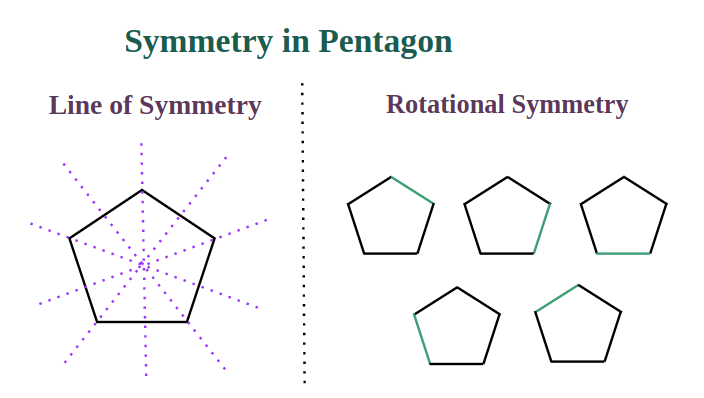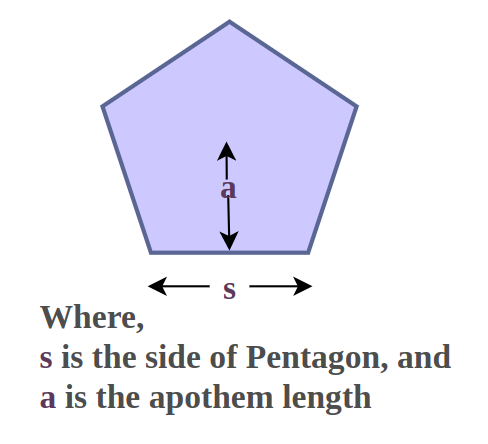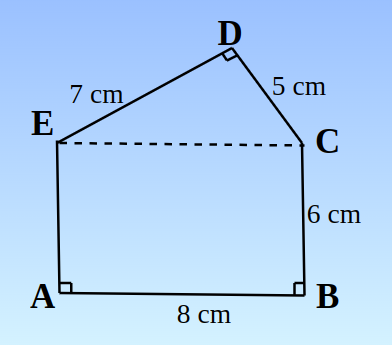Related Articles

# Area of Pentagon

Area of the Pentagon or the area of any polygon is the total space taken by that geometric object. In geometry area and perimeter are the most fundamental quantities of measurement after the side. In general, we study two types of shapes in geometry one is flat shapes(2-D Shapes) and other solid shapes (3-D Forms). We can only calculate the area for the 2-D shapes as for 3-D shapes we need to calculate the surface area. Geometry also deals with the parameters of these shapes and gives standard formulas for determining their parameters like area, perimeter, volume, etc.

This article deals with one of the flat(2-D) shapes named “Pentagon” and gives a brief description of the Pentagon and its properties. This article also explains the method to calculate the area of a pentagon along with some sample problems for better understanding.

## What is a Pentagon?

The word Pentagon denotes ‘five angles’ as it is derived from the Greek words “Penta” which means five and “gonia” which means angles, thus Pentagon is a geometrical shape having five sides and five angles (interior). A regular pentagon has equal five sides, equal five interior angles of measure 108°, and also have five lines of reflectional and rotational symmetry.

In addition to the geometric object pentagon, “Pentagon” also refers to the headquarters of the United States Department of Defence, as this building looks like the Pentagon itself. This building is one of the largest office buildings in the world.

### Examples of Pentagon

There are many examples of the Pentagon either regular or irregular in the daily lives of a common man, some of those examples are as follows:### Properties of a Pentagon

A pentagon is a two-dimensional shape with five sides and five interior angles, with the following properties:

• The sum of all interior angles of a pentagon is 540°.
• For Regular Pentagon:
• All sides are equal.
• All interior angles are equal and have a measure of 108°.
• All exterior angles are also equal and have a measurement of 72°.
• Regular pentagons have five lines of symmetry which divide the pentagon into congruent parts.
• Also, regular pentagons have five rotational symmetries as well.
• It has 5 diagonals meeting at the same point.
• The ratio of the length of its diagonal to the side of the pentagon is always a golden ratio (1 + √5)/2.## Area of Pentagon Formula

For regular pentagons, if the side is represented by s and apothem length which is represented in the following diagram, we can calculate the area of the pentagon using the formula:Area of pentagon = 1/2 × p × a = 5/2 × s × a

## How to find the Area of Pentagon?

There are various ways to find the area of the Pentagon, which are explained as follows:

### Area of Pentagon with Apothem Length

The area of a pentagon is determined by its side and apothem length. The formula of the area of a pentagon is derived by multiplying any side and apothem length by 5/2. Mathematically the formula is given by

Area of Pentagon(A) = (5/2) s × a

Where,

• s is the side
• a is apothem length

For example: If a side of a pentagon is 12cm and its apothem length is 6cm, the area of the pentagon can be determined by

Area of pentagon = (5/2) × side × apothem length

⇒ Area of pentagon= (5/2) × 12 × 6

⇒ Area of pentagon = 180 cm2

### Area of Regular Pentagon

The area of the pentagon can also be calculated only by using the length. If the side of the regular pentagon is s, then the area of the pentagon can be calculated using the following formula:

Area of Pentagon =For example: If a pentagon is given a side length of 5 cm, the area of the pentagon can be determined by

Area of pentagon =⇒ Area of pentagon =⇒ Area of pentagon = 43.01cm2

### Area of Irregular Pentagon

The area of the Irregular Pentagon can be calculated by splitting the pentagon into small triangles of quadrilaterals (whichever is the most efficient according o the problem) and then calculating their individual areas and adding them together to find the area of the irregular pentagon.

Example: Calculate the area of the irregular pentagon shown in the diagram.Solution:

The pentagon ABCDE can be divided into two parts i.e., rectangle ABCE and triangle DCE.

Thus, Area of ABCDE = Area of ABCE + Area of DCE

⇒ Area of ABCDE = AB × BC + 1/2 × CD × DE

⇒ Area of ABCDE = 8 × 6 + 1/2 × 5 × 7

⇒ Area of ABCDE = 48 + 17.5 = 65.5 cm2

## Sample Problems on the Area of the Pentagon

Problem 1. Find the area of a pentagon with a side of 5 cm and an apothem length of 4 cm.

Solution:

Given

Side of pentagon = 5cm

apothem length = 4cm

We have,

Area = (5/2) × s × a

⇒ A = (5/2) × 5 × 4

⇒ A = 50cm2

Problem 2. Find the area of a pentagon with a side of 12cm and an apothem length of 6cm.

Solution:

Given:

Side of pentagon = 12cm

apothem length = 6cm

We have,

Area = (5/2) × s × a

⇒ A = (5/2) × 12 × 6

⇒ A = 180cm2

Problem 3. Find the area of a pentagon with a side length of 4cm.

Solution:

Given:

Side length of pentagon is 4cm

We have,

Area of pentagon =⇒ A =⇒ A = 27.52cm2

Problem 4. Find the area of a pentagon with a side length of 6cm.

Solution:

Given:

Side length of pentagon is 6cm.

We have,

Area of pentagon =⇒ A =⇒ A = 61.93cm2

## FAQs on Area of Pentagon

### Q1: How Many Sides does a Pentagon have?

Pentagons have five sides, five internal angles as well as five vertices.

### Q2: How Many Diagonals are there in a Pentagon?

There are 5 digonals in the geometric object pentagon.

### Q3: How to Find the Area of Pentagon?

We can find area of pentagon using the formula A =(5/2) × s × a, where s is the side length of pentagon and a is the apothem length.

### Q4: How many Triangles are in a Pentagon?

Any polygon can be spilt into unique triangles, where joining them we get the original polygon. Pentagons can be divided into three such triangles.